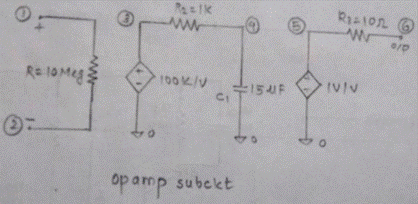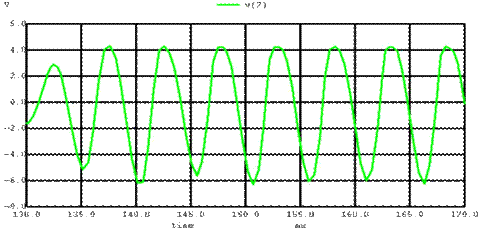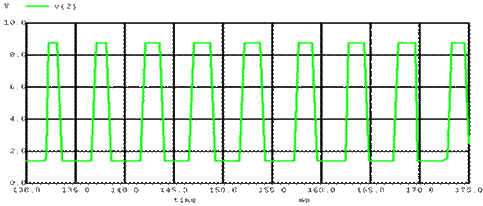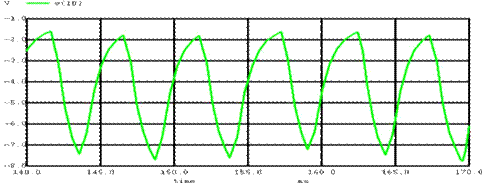Home > mini projects > SIMULATION OF FUNCTION GENERATORSIMULATION OF FUNCTION

GENERATOR

ABSTRACT:

A function generator is an electronic device in which various waveforms are generated and its output can be used as input to other various analog circuits. The waveforms which we tried to generate are sinusoidal , square wave and triangular waves. Any one waveform is generated first and then processing is done on it to obtain other required waveforms.

INTRODUCTION:

A function generator can provide different waveforms. This has an advantage over pure sine wave oscillator as function generator can also produce non-sinusoidal shapes as well.We have taken sinusoidal as the basic wave form generated with the help of "rc-phase shift oscillator". That waveform is passed through a "schmitt trigger" to obtain a square wave. An integrator follows it which gives a triangular wave. Thus the required waveforms are obtained.

RC PHASE SHIFT OSCILLATOR:

We are considering RC PHASE SHIFT by using transistors. The array resistors and capacitor values give required phase shift. The transistor gives a phase shift of 180 and hence the complete RC network to give a phase shift of 180. We have used 3 RC network, each giving a phase shift of 60.

The phase angle is given by Î¦ = tan-1 (Xc/R).

The values are R=10K and C=0.05u and frequency is 200Hz.

SCHMITT TRIGGER:

A schmitt trigger is a positive feedback comparator which holds the output to high as long as the input signal is greater than a reference voltage level ,otherwise it drops to zero. Usually the Schmitt trigger is designed using opamps. The difficulty faced while using opamps was that we approximated it as circuit of R,C and dependent sources. The two voltages "+Vee" and "-Vee" were not taken into account. Hence we switched to a transistor based Schmitt trigger.

WORKING: When an input sine wave is applied, as soon as the input voltage attains a value equal to the sum of the voltages across Rb1 and Re, Q1 turns ON (saturation) and Q2 turns OFF( cut-off). Thus a square wave is produced of Vp-p of 7.3VINTEGRATOR:

An integrator is simulated with the help of the sub circuit of an approximated opamp. An op-amp integrating circuit produces an output voltage which is proportional to the area (amplitude multiplied by time) contained under the waveform. An ideal op-amp integrator uses a capacitor connected between the output and the op-amp's inverting input terminal.

Thus the pulse waveform gets integrated and we obtain a triangular waveform. The formula used is:CIRCUIT DIAGRAM:MAIN CODE*function generator*

.model bjt npn

.model bit pnp

.include opamp.cir

Q4 16 13 18 bjt

R11 13 0 20k

R12 13 0 10k

R13 14 0 10k

R14 15 0 10k

C11 13 14 0.05u

C12 14 15 0.05u

C13 15 16 0.05u

C14 16 7 10u

R15 13 19 100k

R16 16 19 4.7k

R17 18 0 680

C15 18 0 10u

R18 7 0 10k

Vcc1 19 0 dc 32V

Q1 5 6 4 bjt

Q2 2 3 4 bjt

r1 7 6 3.3k

r2 1 5 6k

r3 1 2 14k

r4 5 3 50k

r5 3 0 25k

r6 4 0 2k

r7 2 8 100k

r8 8 10 100k

r9 9 0 100k

Cf 8 10 0.01u

xop 8 9 10 opamp

c1 5 3 10f

Q3 12 11 0 bjt

r10 10 11 1k

r21 1 12 10k

vcc 1 0 dc 10

.control

tran 0.5m 500m

run

plot v(10)

plot v(2)

plot v(7)

.endc

.endCODE:

SUB-CIRCUIT OF OP- AMP*opamp*

.subckt opamp 1 2 6

R1 1 2 10Meg

R2 3 4 1K

R3 5 6 10

C1 4 0 15u

E1 3 0 1 2 100K

E2 5 0 4 0 1

.endsSIMULATION:

1)SINE WAVE:2)SQUARE WAVE:3)TRIANGULAR WAVE: